PH, Buffer Solutions, Solubility & Solubility Product

# PH, Buffer Solutions, Solubility & Solubility Product Notes | Study Physical Chemistry - Chemistry

## Document Description: PH, Buffer Solutions, Solubility & Solubility Product for Chemistry 2022 is part of Physical Chemistry preparation. The notes and questions for PH, Buffer Solutions, Solubility & Solubility Product have been prepared according to the Chemistry exam syllabus. Information about PH, Buffer Solutions, Solubility & Solubility Product covers topics like and PH, Buffer Solutions, Solubility & Solubility Product Example, for Chemistry 2022 Exam. Find important definitions, questions, notes, meanings, examples, exercises and tests below for PH, Buffer Solutions, Solubility & Solubility Product.

Introduction of PH, Buffer Solutions, Solubility & Solubility Product in English is available as part of our Physical Chemistry for Chemistry & PH, Buffer Solutions, Solubility & Solubility Product in Hindi for Physical Chemistry course. Download more important topics related with notes, lectures and mock test series for Chemistry Exam by signing up for free. Chemistry: PH, Buffer Solutions, Solubility & Solubility Product Notes | Study Physical Chemistry - Chemistry
 1 Crore+ students have signed up on EduRev. Have you?

17. Calculation of pH of Acidic Buffer Solution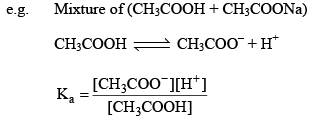Since most of CH3COO comes from salt (CH3COONa) and hence conc. of CH3COO will be conc. of CH3COONa.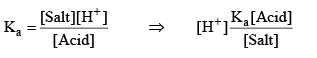Taking (–ve) log both side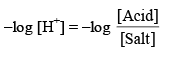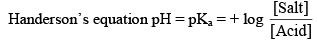Illustration: Find pH of solution contains 0.1 M (100 ml) CH3COOH and mixed with 0.1 M, 50 ml NaOH. Ka = 10–5.

Solution: Here, an acid and base are mixed. So, first of all, Acid–Base react ion take place.

MIlimoles of CH3COOH = 10 mmol

Milimoles of NaOH = 5 mmol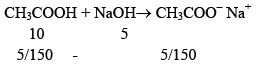Now, solution is having weak acid CH3COOH and it’s salt having common ion. So, this solution is buffer solution ⇨ Acidic Buffer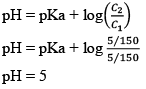18. Basic Buffer

A mixture containing equimolar solutions of ammonium hydroxide and its almost completely dissociated salt, ammonium chloride, constitutes another good basic buffer.The mixture contains undissociated NH4OH as well as NH4+ and Cl ions. The buffer action of this mixture many now be considered. If a strong acid is added. The H+ ions added are neutralized by the base NH4OH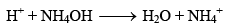If a strong base is added, the OH ions added are neutralized by NH4+ ions forming very slightly dissociated NH4OH.

19. Calculation of pH of Basic Buffer Solution

e.g. Mixture of (NH4OH + NH4Cl)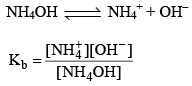Since most of NH4+ ions comes salt (NH4Cl) so we take NH4+ conc. of salt (NH4Cl).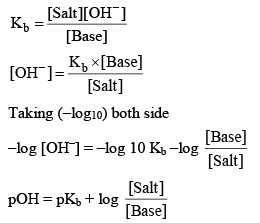Hence pH = 14 –pOH at 14°C, Kw = 1 × 10–14 mol/litre.

Illustration:0.1 M, 100 ml NH4OH is titrated with 0.1 M, H2SO4 solution. Calculate the pH if kb = 10–5

(a) Nothing is added

(b) 25 ml, H2SO4 is added

(c) 0.1 M, 20 ml NaOH is mixed in resulting solution for made in part b.

(d) Find the pH if 0.1M, 10 ml H2SO4 is added in part b.

Solution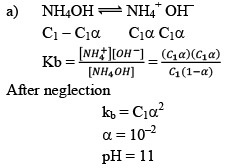b) When an acid H2SO4 is added with NH4OH (a base), Acid-Base reaction takes place.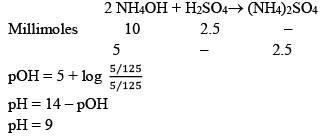c) In part b we are having a weak base and its salt when NaOH is added it will React with (NH4)2SO4.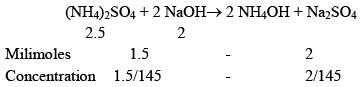Now, the solution is having weak base and it’s salt, hence Buffer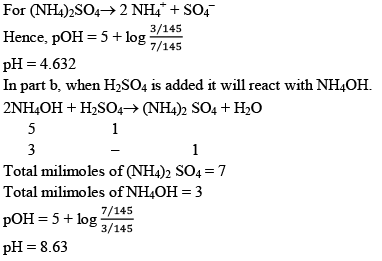20. Salt Buffer

A salt buffer is a solution of a salt which itself can act as a buffer. Such salt is the salt of a weak acid and weak base.

For example,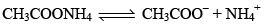When an acid is added, it reacts with CH3COO to produce CH3COOH and when a base is added, it reacts with NH4to produce NH4OH.

21. Buffer Capacity or Buffer Index

Buffer capacity of a solution is defined in terms of buffer index which is the change in the concentration of Buffer acid (or base) required for change of it’s pH value by one, keeping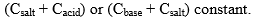Let there be a buffer solution of volume 1 litre with ‘x’ mole of acid and ‘S’ moles of ‘salt’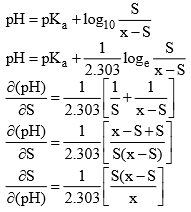Maximum value of Buffer Index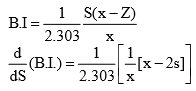For maximum value of Buffer index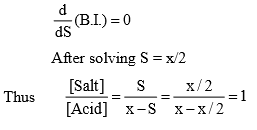Hence max. value of Buffer index occurs when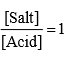22. Buffer Range

It is difficult to give an exact limit into which a buffer can be used it in generally accepted that a solution has useful buffer capacity provided that the value of [Salt]/[Acid] lie within the range of 10 to 0.1. Hence from Henderson equation.

pKa + log 0.1 < pH < pKa + log10 10

pKa – 1 < pH < pKa + 1

Outside this range the Buffer capacity it too small to be of any practical application.

23. Hydrolysis of Salt

Salts are strong electrolytes when dissolved in water, they dissociated almost completely into cations or anions. If anion reacts with water it is called as anionic hydrolysis.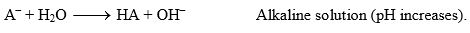If cation reacts with water it is called as cationic hydrolysis.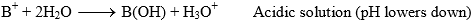“The phenomenon of the interaction of anions and cations of the salt with H+ and OH ions furnished by water yielding acidic or alkaline solution is known as salt hydrolysis.

For the study of hydrolysis salts are divided into 4 groups.

Hydrolysis of salt of strong Acid or weak base: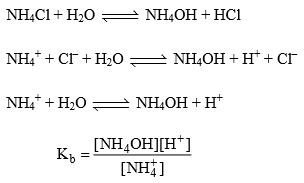Relation B/w Kh, Kb and Kw: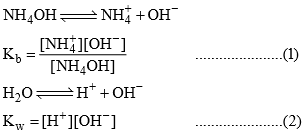Dividing (2) ÷  (1)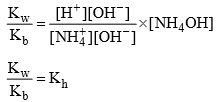Degree of hydrolys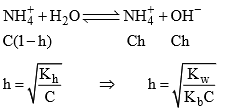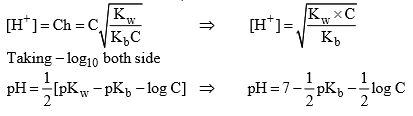Hydrolysis of salt of weak acid and strong base:

CH3COONa is a salt of weak acid (CH3COOH) and strong base (NaOH). After hydrolysis resultant solution will be basic due to presence of strong base (NaOH)/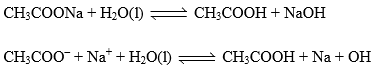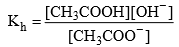Relation between, Kh, Kw and Ka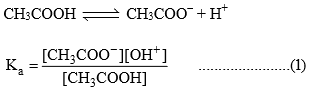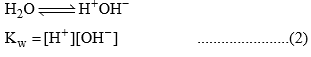Dividing equation (2) ÷ (1)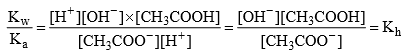Degree of Hydrolysis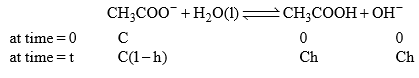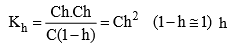should be smaller than 0.1 than 1 – h = 1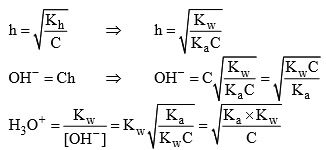Taking (- ve) log both side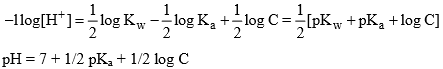pH will be more than 7, hence resultant solution will be basic in nature.

Hydrolysis of salt of Weak Acid and Weak Base:

Let’s take the salt CH3COONH made of salt of weak acid (CH3COOH) and Weak base (NH4OH).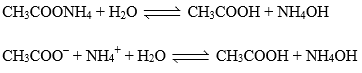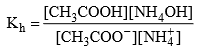Relation between, Kh, Kw, Ka and Kb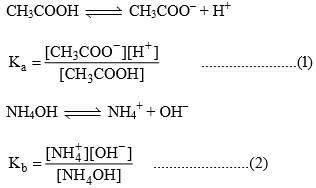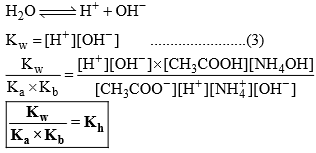Degree of Hydrolysis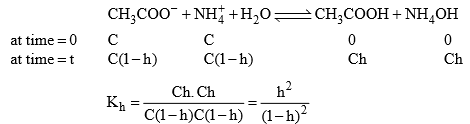If  h ≤ 0.1,  1 – h ≌ 1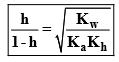The acetic acid formed would partially decompose to give CH3COO and H+. But because of common ion effect (that is, due to the unhydrolyzed CH3COO) it is possible to neglect the acetate ion coming from CH3COOH.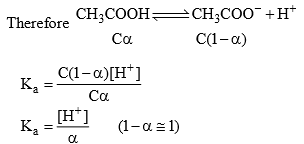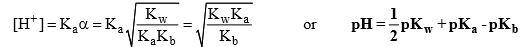This expression is independent of conc. of the salt.

(i) if Ka = Kb, pH = 7 solution will be neutral

(ii)  if Ka > Kb, pH < 7, acidic solution

(iii) if Ka < Kb then pH > 7, alkaline solution

In the hydrolysis of salt of weak acid and a weak base such as NH4CN, CH3COONH4. Both the ions are hydrolyzed, if we assume Ka ≌ Kb, then the hydrolysis of the cation and anion of the salt occur approximately to equal extent for a salt which has Ka < Kb. it would be expected at the first glance that CN ions hydrolyzed to a much greater extent than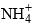ions. However, the hydrolysis of CN ion produced OH ions according to the equation.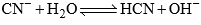Which can react with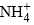ions as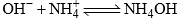This latter reaction causes equilibrium in the from reaction to be displaced to the right. Because OH ions are removed from the solution. Also the production of OH by the former reaction displaces the latter reaction to the right. Therefore the hydrolysis of one ion drags the hydrolysis of the other ion along so that both the hydrolysis are fairly extensive far in extant from each other so it is fairly safe to assume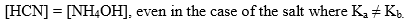Illustration: What is the pH of 0.4 M aqueous solution of NaCN, given that the pkb (CN) is 70.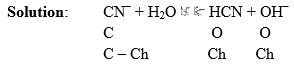pKb(CN) is given means equilibrium constant of this reaction is given. This reaction expresses the basicity of CN ion.

pKb = - log Kb

4.7 = - log Kb

Kb = 1.9 × 10–5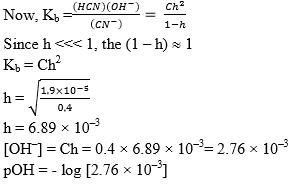pOH = 2.55

pH = 11.45

Illustration: Find out the Kh of a centimolar solution of NH4Cl. If the dissociation constant for NH4OH is 10–6 and Kw = 10–14. Find the degree of hydrolysis and pH of solution.

Solution: NH4Cl is a salt of strong acid and weak base.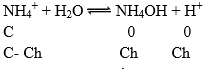Equilibrium constant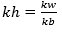Kb = 10–6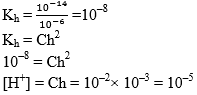pH = 5

24. Case IV: Salts of Strong Acid + Strong Base

e.g., NaCl, KNO3,………..etc.

This category of salts not undergo salt hydrolysis

25. Solubility and Solubility Product

A solution which remain in contact with excess of the solute is said to be saturated. The amount of a solute, dissolved in a given volume of a solvent (in 1 litre) to forma saturated solution at a given temperature, it termed as the solubility of the solute in the solvent at that temperature.

Molar Solubility:

No. of moles of solute dissolved in per litre of solution

Solubility Product:

In a saturated of a salt, there exists a dynamic equilibrium between the excesss of the solute and ions furnished by that parts of the solute which has gone in solution. The solubility product of a sparingly soluble salt is given as product of the conc. of the ions raised to the power equal to the no. of times the in occur in the equation after the dissociation of the electrolyte.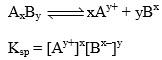Let the solubility of AxBy is S then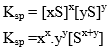The principle of solubility product is applicable for sparingly soluble salt.

26. Common-ion Effect on Solubility

The common ion present in the solution decrease the solubility of a given compound e.g. The solubility of BaSO4 in Na2SO4 solution is smaller than in an aqueous solution.

Consider saturated solution of AgCl. If a salt having either of the ion common to AgCl say KCl is added to it, then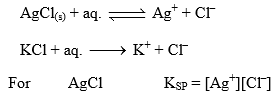[Cl] Increases in solution due to presence of KCl and thus to have KSP constant, [Ag+] will decrease or AgCl will precipitate out from solution, i.e., solubility of AgCl will decrease with increasing concentration of KCl in solution.

Let 0.1 M KCl(aq.) solution with AgCl(aq.). If solubility of AgCl is s mol litre–1, then

For AgCl               KSP = [Ag+][Cl

KSP = s(s + 0.1)

s being small in comparison to 0.1 and thus may be neglected therefore,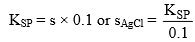Where s is solubility of AgCl in presence of 0.1 M KClaq.

27. Ionic Product

For a solution of a salt at a specified concentration, the product of the concentration of the ions, each raised to the proper power, is called as the ionic product for a saturated solution in equilibrium with excess of solid, the ionic product is equal to solubility product.

At equilibrium, ionic product = solubility product

If ionic product is less than solubility product it means solution is unsaturated means more salt can be dissolve in it.

If ionic product greater than solubility it means solution is holding more salt than can dissolve in it, therefore ppt started till, until or unless ionic product becomes equal to Ksp

The document PH, Buffer Solutions, Solubility & Solubility Product Notes | Study Physical Chemistry - Chemistry is a part of the Chemistry Course Physical Chemistry.
All you need of Chemistry at this link: Chemistry

## Physical Chemistry

67 videos|106 docs|31 tests
 Use Code STAYHOME200 and get INR 200 additional OFF

## Physical Chemistry

67 videos|106 docs|31 tests

Track your progress, build streaks, highlight & save important lessons and more!

,

,

,

,

,

,

,

,

,

,

,

,

,

,

,

,

,

,

,

,

,

,

,

,

,

,

,

;